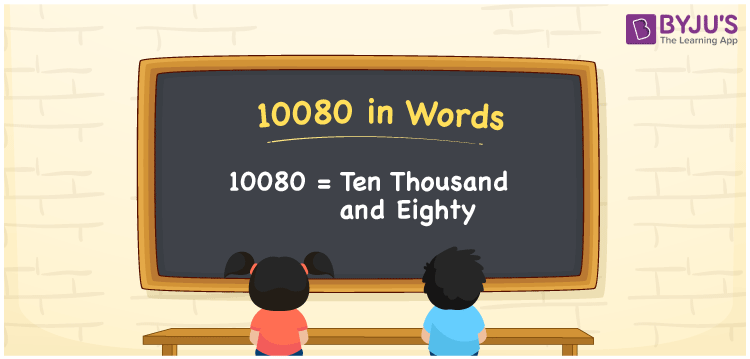In English, the number 10080 is written as Ten Thousand and Eighty. For example, if you paid Rs. 10080 for groceries this month, you can say, “I paid Rupees Ten Thousand and Eighty for groceries this month”. Writing numbers in word form is not difficult if we use a place value chart. 10080 is a cardinal number as it denotes a specific quantity. Let us learn how to convert the number 10080 into words in this article.

 10080 in Words Ten Thousand and Eighty Ten Thousand and Eighty in numerical form 10080

## 10080 in English Words

English alphabet is used to write numbers in words in Mathematics. Thus, 10080 is written in words as Ten Thousand and Eighty.## How to Write 10080 in Words?

We use a place value chart to represent the number 10080 in word form. Since 10080 is a five-digit number. So, let us create a chart with five columns.

 Ten-Thousands Thousands Hundreds Tens Ones 1 0 0 8 0

Hence, we can write the expanded form as:

1 x Ten Thousand + 0 x Thousand + 0 x Hundred + 8 x Ten + 0 x One

= 1 x 10000 + 0 x 1000 + 0 x 100 + 8 x 10 + 0 x 1

= 10000 + 0 + 0 + 80 + 0

= 10000 + 80

= 10080

= Ten Thousand and Eighty

Therefore, 10080 in words is written as Ten Thousand and Eighty

Interesting way of writing 10080 in words

1 = One

10 = Ten

100 = One Hundred

1008 = One Thousand Eight

10080 = Ten Thousand and Eighty

Thus, the word form of the number 10080 is Ten Thousand and Eighty

10080 is a natural number that is the successor of 10079 and the predecessor of 10081

• 10080 in words – Ten Thousand and Eighty
• Is 10080 an odd number? – No
• Is 10080 an even number? – Yes
• Is 10080 a perfect square number? – No
• Is 10080 a perfect cube number? – No
• Is 10080 a prime number? – No
• Is 10080 a composite number? – Yes

## Frequently Asked Questions on 10080 in Words

Q1

### How to write 10080 in words?

10080 in words is written as Ten Thousand and Eighty.
Q2

### What is the place value of 8 in 10080?

The place value of 8 in 10080 is Tens, and its value is 80.
Q3

### Is 10080 an even number?

Yes, 10080 is an even number since it is divisible by 2.
Test your Knowledge on 10080 in Words